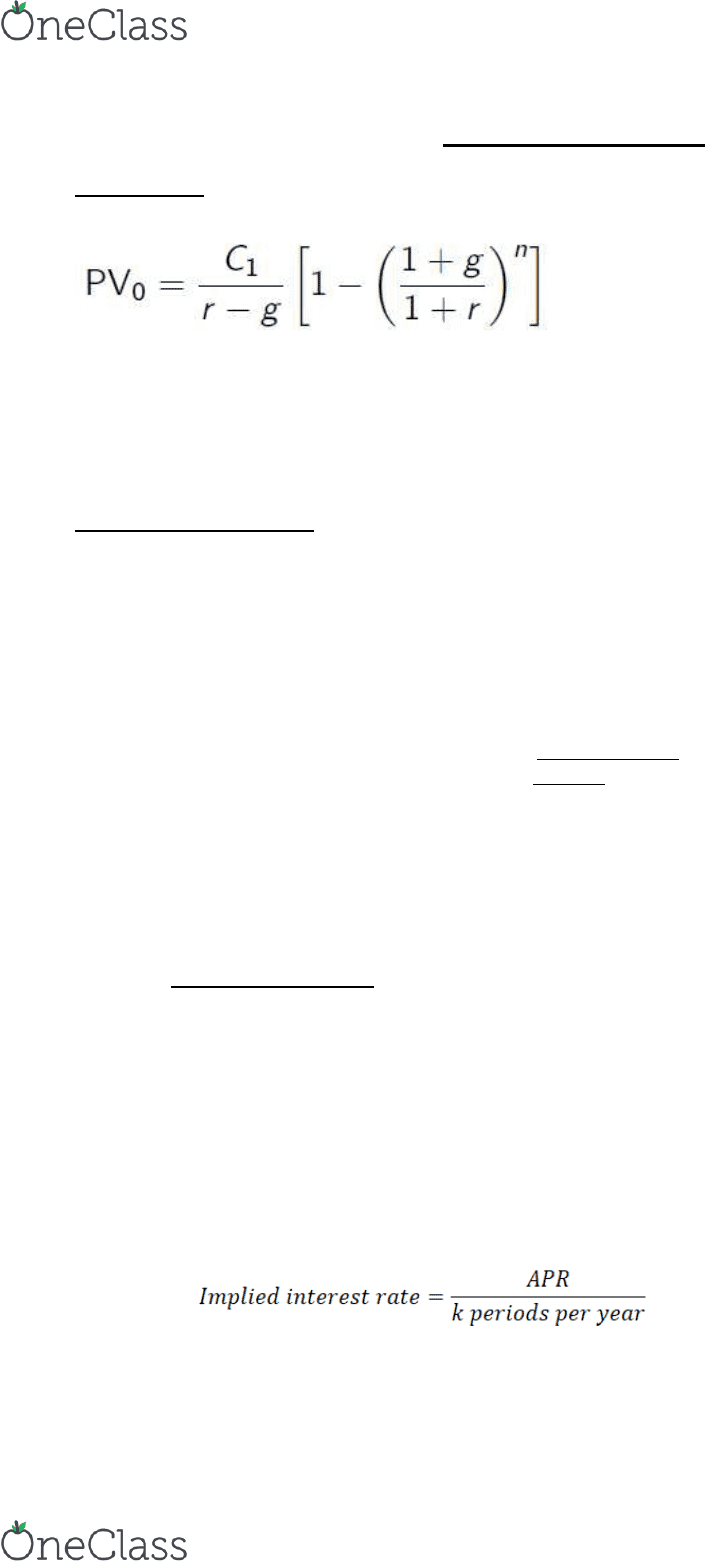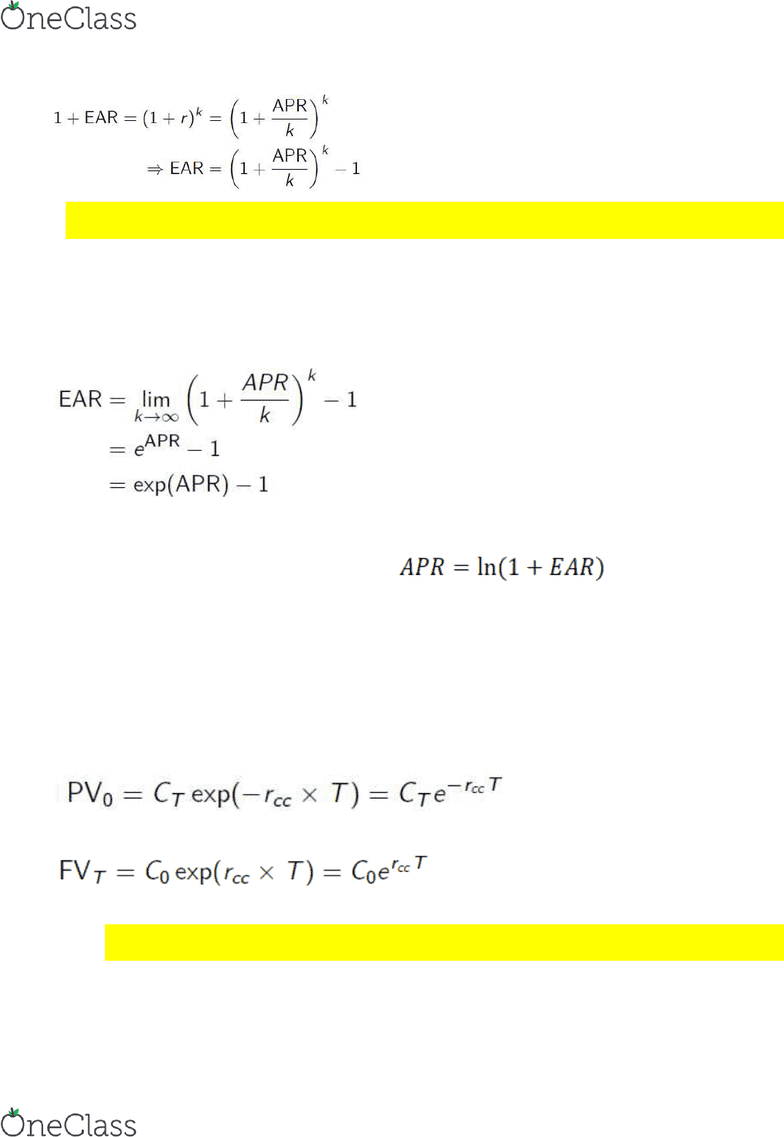# AFM273 Chapter Notes - Chapter 5: Net Present Value, Annual Percentage Rate, Real Interest Rate

66 views7 pages1
Ch. 5 Interest Rates
Introduction
Recall the formula for the present value of a growing annuity with n payments, the first being C1:
As emphasized earlier, this formula assumes that the first payment is one period from now and
that both r and g are measured in terms of a period
We often have to adjust r (and sometimes also g) in order to correctly calculate present or future
values
This requires an understanding of how interest rates are quoted in practice
Effective Annual Rates
Effective annual rate (EAR): indicates the total amount of interest earned over a 1-year period
Adjusting the EAR to an Effective Rate over Different Periods of Time
If r is the effective rate for one period, then
1 + Equivalent n-Period Effective Rate = (1 + r)n
Equivalent n-Period Effective Rate = (1 + r)n 1
o If n > 1, this is an effective rate over more than one period
o If n < 1, it is an effective rate over a fraction of a period
Annual Percentage Rates
Annual percentage rate (APR): indicates the amount of simple interest earned over a 1-year
period
Interest rates quoted by banks are APRs
The APR ignores the effect of compounding, even though compounding may occur
If compounding does happen, then the APR will not give the correct amount of interest unless it is
first converted to an EAR
o APR < actual amount of interest earned
In such cases, we cannot simply use the APR to calculate present or future values
To convert to an EAR, we have to know the compounding frequency (annual, semiannual, monthly,
etc.)
The APR with k compounding periods is actually just an indirect way of quoting the effective rate
r earned each compounding period
The APR with k compounding periods is actually just an indirect way of quoting the effective rate
earned each compounding period
APR itself cannot be used as a discount rate and it is not an EAR
In particular, the implied effective interest rate per compounding period r is given by APR /(k
periods per year)
We can then convert to an EAR using
find more resources at oneclass.com
find more resources at oneclass.com
Unlock document

This preview shows pages 1-2 of the document.
Unlock all 7 pages and 3 million more documents.2
Example: if a bank quotes a rate of 4% per year with quarterly compounding, what is the EAR?
What if instead the quoted rate is 9% compounded monthly?
Continuous Compounding
EAR increases as the frequency of compounding increases (Table 5.1, p. 142)
In principle, we can compound as often as we like (every month, every week, every day, every
hour, every second, . . . )
An infinite number of compounding periods in a year corresponds to continuous compounding,
and in this case
If we know the EAR, we can rearrange the expression above to solve for the APR with continuous
compounding:
Continuously compounded rates are not often seen directly, but they are often used behind the
scenes in some areas of finance (e.g. fixed income, options) because some calculations are much
simpler with continuous compounding
Present and Future Values with Continuous Compounding
We will only consider the case with a single cash flow
Denote the continuously compounded APR as rcc
o If CT is to be received T years from today, then
o If C0 is invested today, then
Suppose that the continuously compounded interest rate rcc = 5%
o What is the present value of a bond paying \$1,000 4.5 years from today?
o What is the future value 8 years and 9 months from now of an investment of \$250 today?
DO NOT READ APPENDIX (ANNUITIES AND PERPETUITIES)
find more resources at oneclass.com
find more resources at oneclass.com
Unlock document

This preview shows pages 1-2 of the document.
Unlock all 7 pages and 3 million more documents.